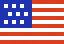New Swift-BAT/GUANO and IceCube Notice Types Available! See news and announcements

# GCN Circular 7812

Subject
Konus-Wind observation of GRB 080603B
Date
2008-06-04T17:04:26Z (15 years ago)
From
Valentin Pal'shin at Ioffe Inst <val@mail.ioffe.ru>
``````S. Golenetskii, R.Aptekar, E. Mazets, V. Pal'shin, D. Frederiks,
and T. Cline on behalf of the Konus-Wind team report:

The long GRB 080603B (Swift-BAT trigger #313087: Mangano et al., GCN
7794, Tueller et al., GCN 7806) triggered Konus-Wind at T0=70692.383 s
UT (19:38:12.383).

The burst light curve shows several pulses with a total duration of ~70s.

As observed by Konus-Wind the burst
had a fluence of 4.50(-0.90, +1.53)x10^-6 erg/cm2,
and a 256-ms peak flux measured from T0+0.624 s
of 1.51(-0.38, +0.40)x10^-6 erg/cm2/s
(both in the 20 keV - 1 MeV energy range).

The time-integrated spectrum of the burst
(from T0 to T0+73.984 s) can be fitted (in the 20-500 keV range)
by a power law with exponential cutoff model:
dN/dE ~ (E^alpha)*exp(-E*(2+alpha)/Ep)
with alpha = -1.23(-0.54, +0.75),
and Ep = 102(-28, +119) keV (chi2 = 50.5/42 dof).
Fitting by GRBM (Band) model yields:
the low-energy photon index is alpha = -0.94(-0.75, +1.21),
the high energy photon index beta < -1.96,
the peak energy Ep =  85(-30, +91) keV (chi2 = 49.7/41 dof).

The spectrum of the most intense part (from T0 T0+16.640 s)
is well fitted (in the 20 keV - 1 MeV range)
by a power law with exponential cutoff model
with alpha =  -1.20(-0.32, +0.26)
and Ep = 200(-56, +131) keV (chi2 = 63.5/56 dof).
The fluence of this part is 3.51(-0.58, +0.69)x10^-6 erg/cm2.

All the quoted errors are at the 90% confidence level.

Assuming z = 2.69 (Fynbo et al., GCN 7797) and a standard
cosmology model with H_0 = 70 km/s/Mpc, Omega_M = 0.27, Omega_\Lambda =
0.73, the isotropic energy release is E_iso ~7.7x10^52 erg,
the maximum luminosity is (L_iso)_max ~9.4x10^52 erg/s, and
Ep_rest ~350 keV.

The Konus-Wind light curve of this GRB is available